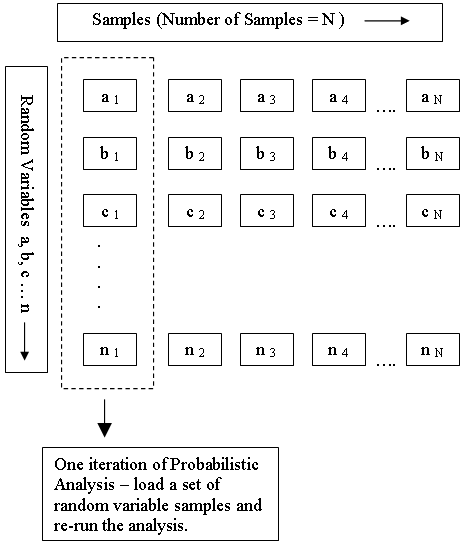RSWebinar: Designing Cost-Effective Ground Improvement Solutions with Settle3 | June 7th, 2023 Register Now

# Probabilistic Analysis Overview

In a traditional slope stability analysis, it is assumed that the values of all model input parameters are exactly known. For a given slip surface, a single value of safety factor is calculated. This type of analysis can be referred to as a DETERMINISTIC analysis.

For most real-world slope stability problems, the values of many input parameters are NOT very well known; therefore a PROBABILISTIC approach to the analysis of slope stability can be useful. In a probabilistic slope stability analysis, the user may assign statistical distributions to model input parameters, such as material properties, support properties, loads, water table location, etc.

By assigning a statistical distribution to one or more model input parameters, this allows the user to account for the degree of uncertainty in the value of the parameters. Input data samples are randomly generated, based on the user-defined statistical distributions. A given slip surface may then have many different values of safety factor calculated. This results in a distribution of safety factors, from which a PROBABILITY OF FAILURE for the slope can be calculated.

The general procedure for carrying out a Probabilistic Analysis with Slide2, is as follows.

## Project Settings

Select the Statistics page in the Project Settings dialog, and select the Probabilistic Analysis checkbox. You may select the Sampling Method, Number of Samples, and Probabilistic Analysis Type. For details about these options, see the Probabilistic Analysis topic in the Project Settings section of this Help system.

## Statistics Menu ( Defining Random Variables )

A Statistics menu will now be available in the Slide2 main menu. The options in the Statistics menu, allow you to select model input parameters (e.g. material properties, support properties, loads etc), and define them as random variables. This is done by selecting a statistical distribution for each selected parameter (random variable), and entering the appropriate parameters for the distribution (standard deviation, minimum and maximum values, correlation coefficient).

In order to carry out a Probabilistic Analysis, you must define at least ONE of your model input parameters, as a Random Variable. See the Random Variables topic for more information.

## Compute

After you have defined all of the desired Random Variables for the Probabilistic Analysis, select Compute. When you select Compute:

• the regular (DETERMINISTIC) slope stability analysis, is always carried out FIRST.
• the Probabilistic Analysis will then automatically follow, as described below.

## How are the Random Variables used in the Analysis?

Each input parameter that is defined as a Random Variable, is sampled according to the statistical distribution you have defined for the variable, the Sampling Method and the Number of Samples (chosen in Project Settings). This generates N values of each Random Variable (where N = Number of Samples).

As shown in the following diagram, each iteration of the Probabilistic Analysis is carried out by loading a new set of random variable samples, and re-running the analysis. This is repeated N times where N = Number of Samples.

## Random Variable Samples used in Probabilistic Analysis• If the Probabilistic Analysis Type (in the Project Settings dialog) = Global Minimum, then the analysis is only carried out on the Global Minimum surface which has been located by the Deterministic analysis.
• If the Probabilistic Analysis Type = Overall Slope, then the entire slip surface search is repeated N times (where N = Number of Samples). In this case, each search iteration may locate a different Global Minimum slip surface.
Any model input parameter that is NOT defined as a Random Variable, is assumed to be "exactly" known and is not involved in the statistical sampling process.

For further details about the Probabilistic Analysis Type in Slide2, see the Probabilistic Analysis topic in the Project Settings section of this Help system.

## Interpret

The results of the Probabilistic Analysis are viewed in the Slide2 Interpret program. A Statistics menu will be available with all of the options for viewing the Probabilistic Analysis results, including histograms, cumulative plots, scatter plots, and other options.